# RD Sharma Solutions for Class 9 Maths Chapter 19 Surface Area and Volume of A Right Circular Cylinder Exercise 19.2

Class 9 Chapter 19 – Surface Area and Volume of A Right Circular Cylinder Exercise 19.2 solutions are provided here. The RD sharma class 9 Maths Solutions are considered as one of the best tools to prepare for the examinations. All exercise 19.2 questions are solved in such a manner that it helps students to understand the concepts with ease. Students are advised to follow the RD sharma Class 9 Solutions while practicing to prepare Maths more effectively.

## Download PDF of RD Sharma Solutions for Class 9 Maths Chapter 19 Surface Area and Volume of A Right Circular Cylinder Exercise 19.2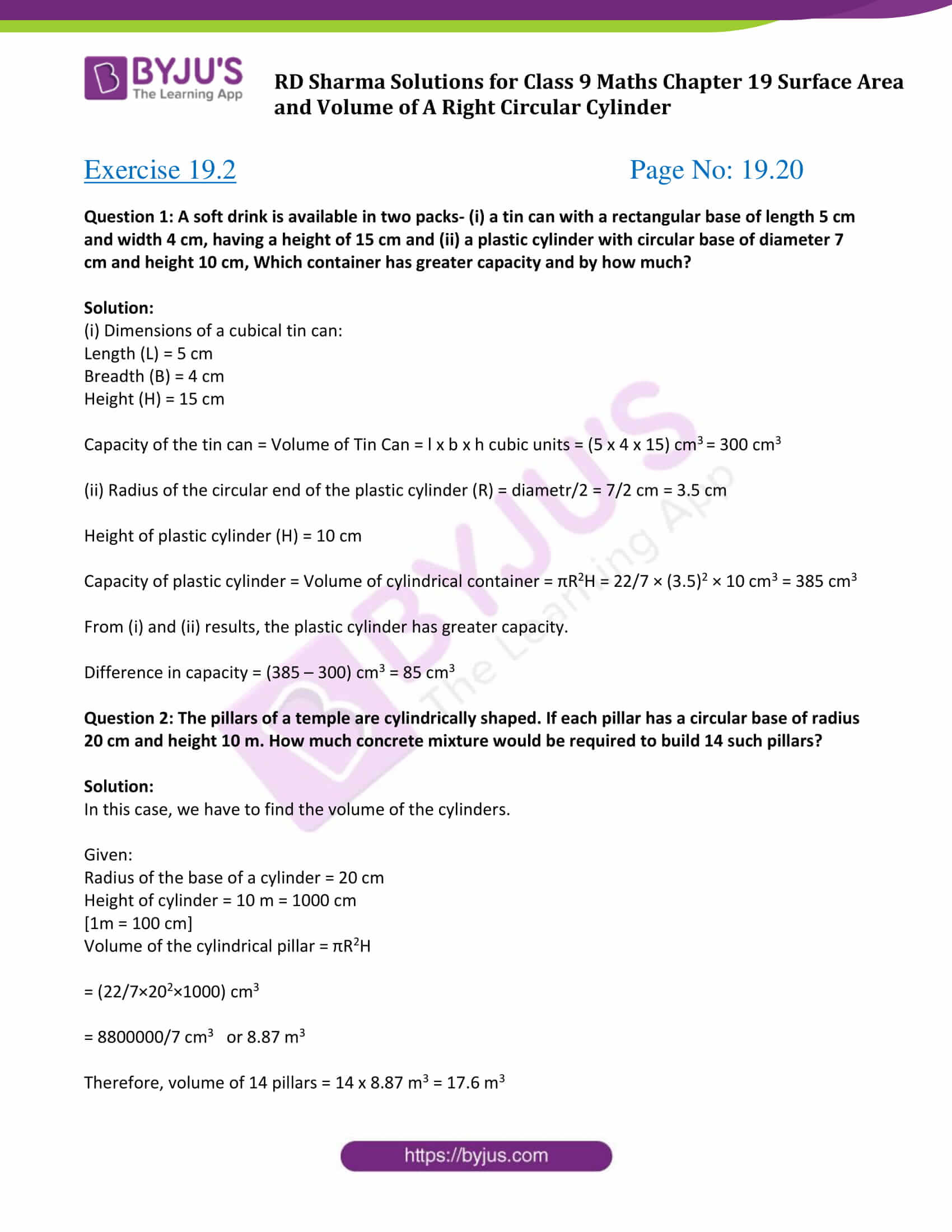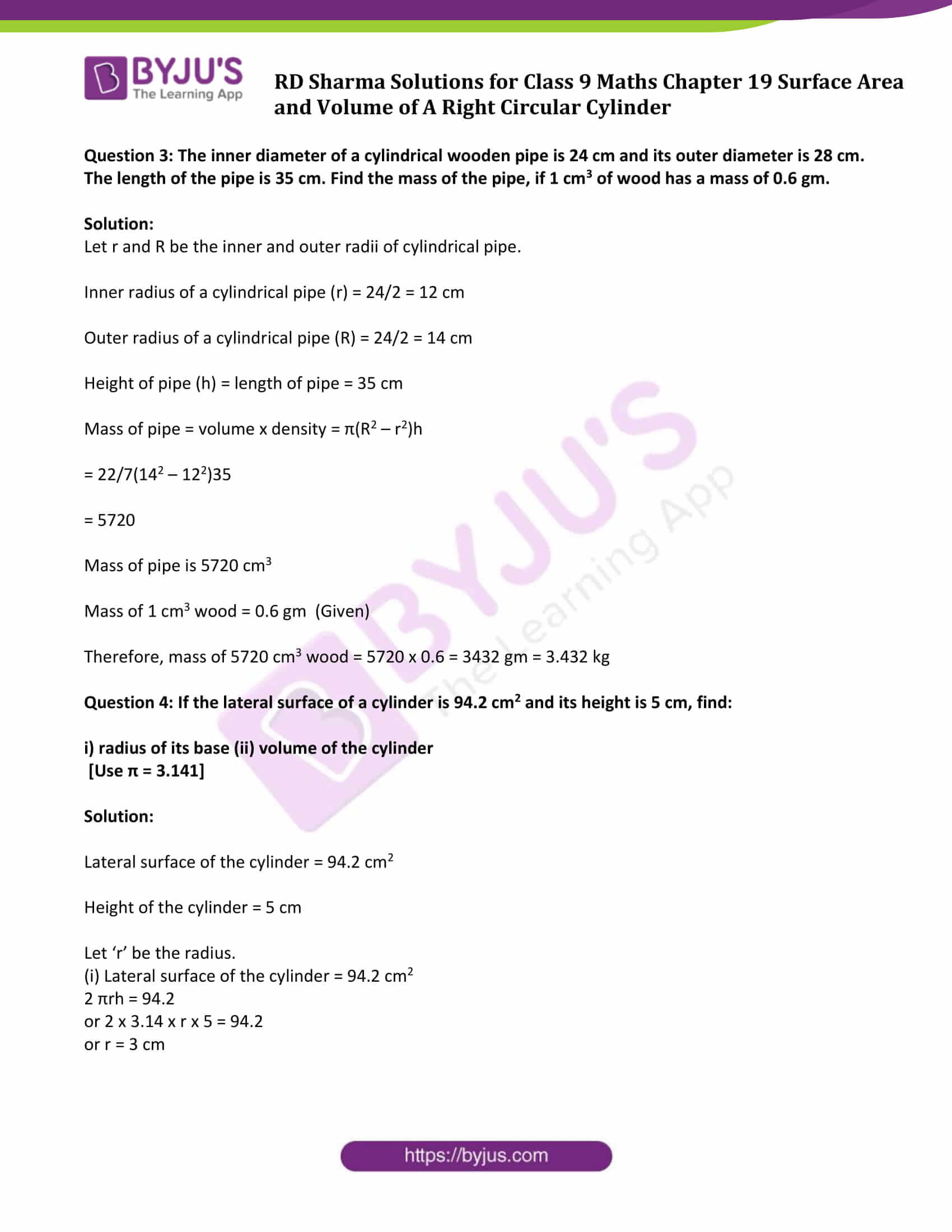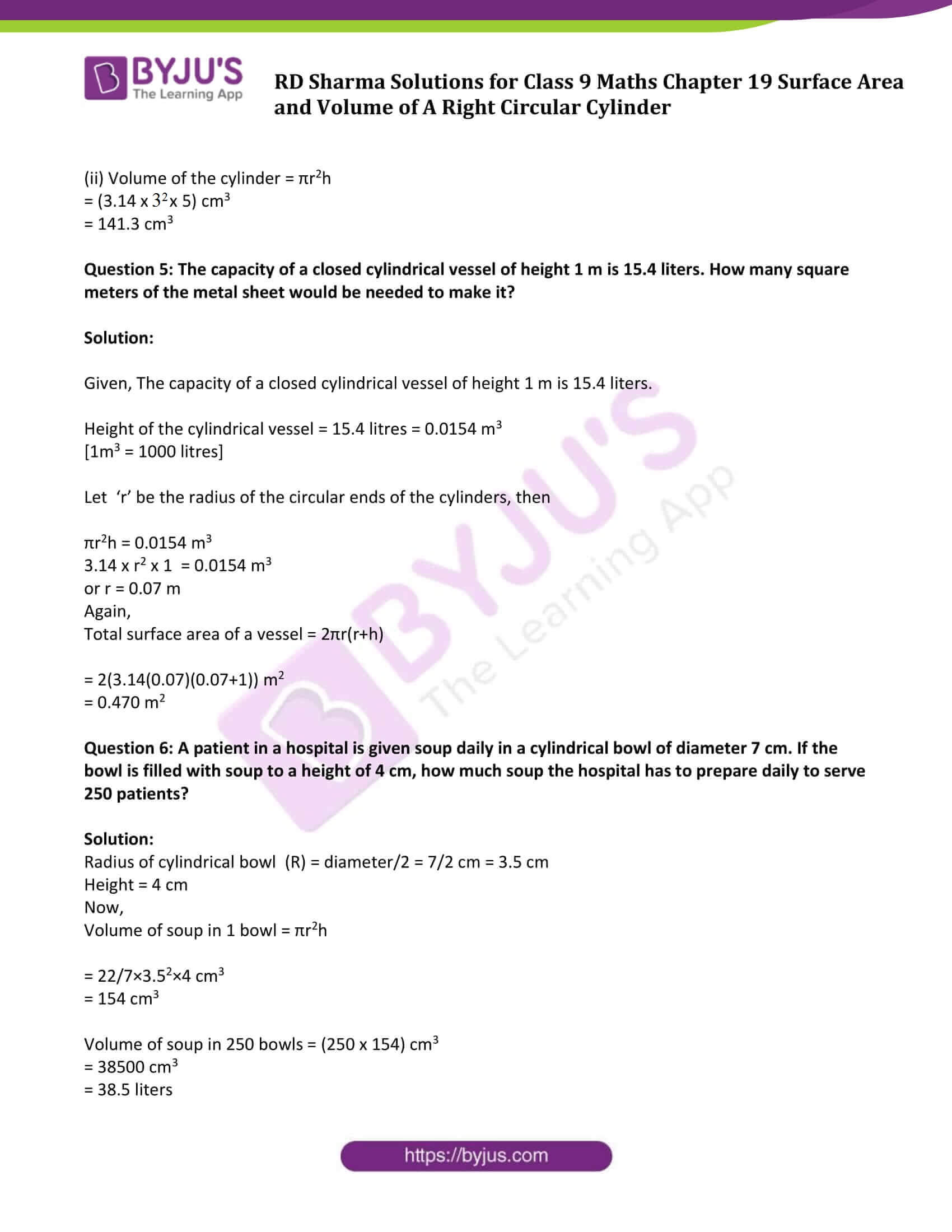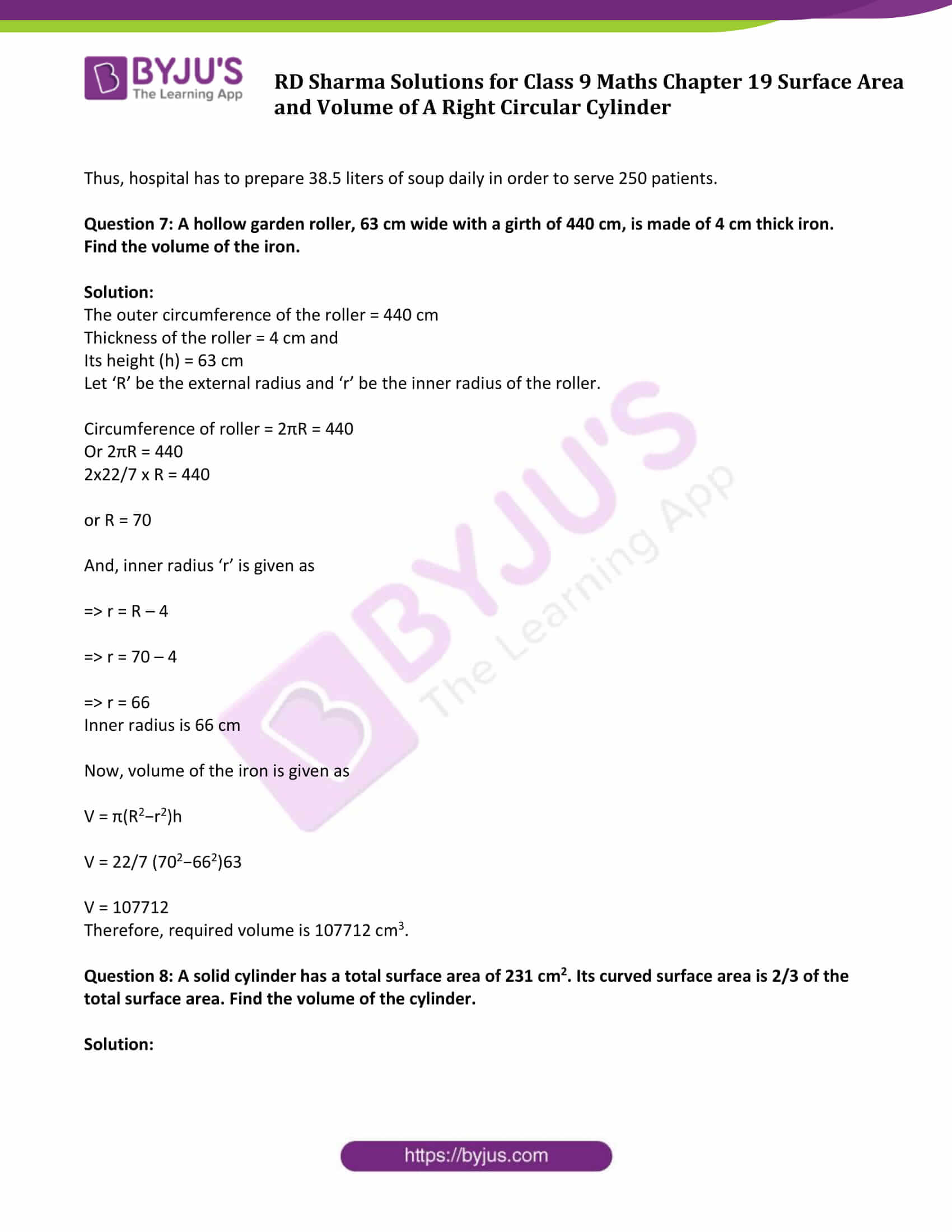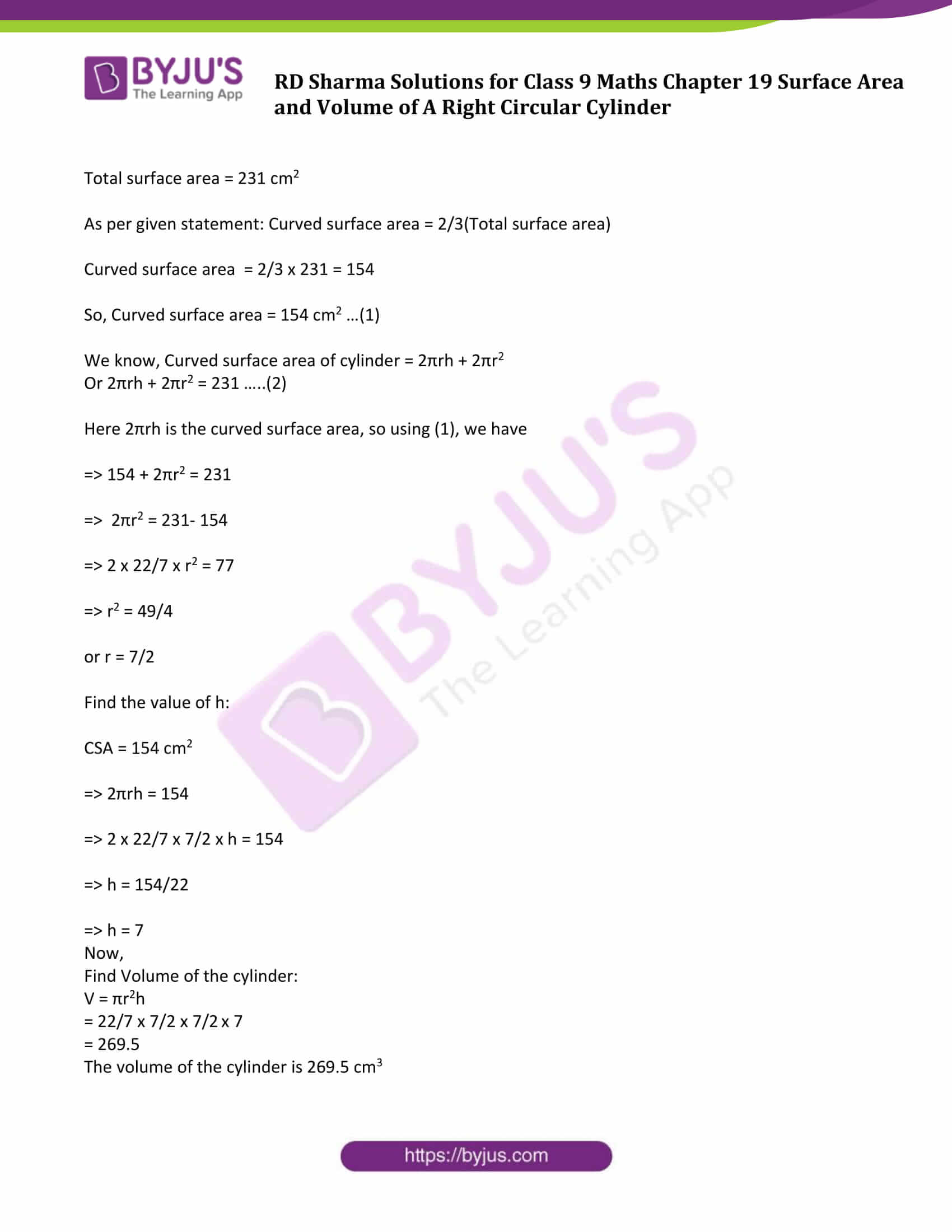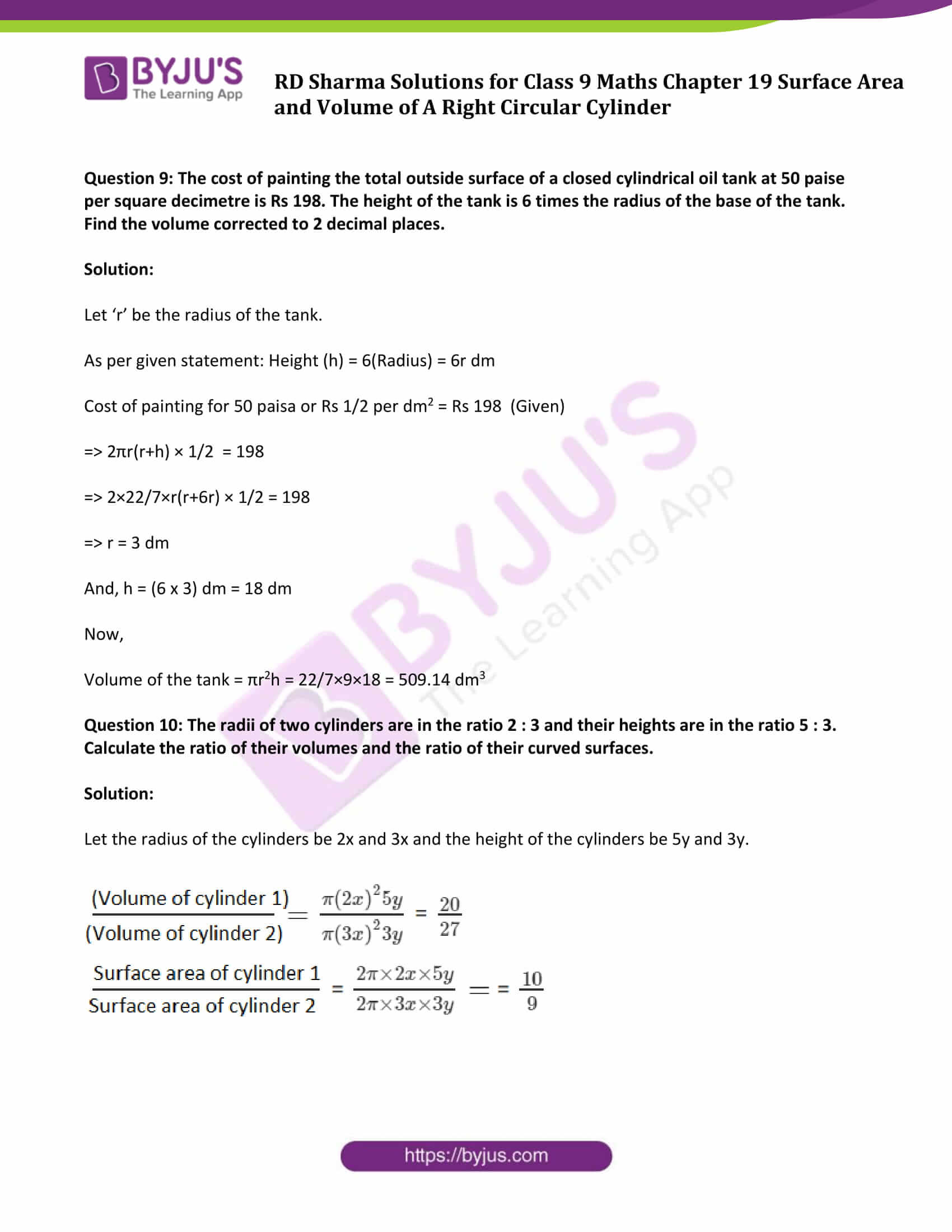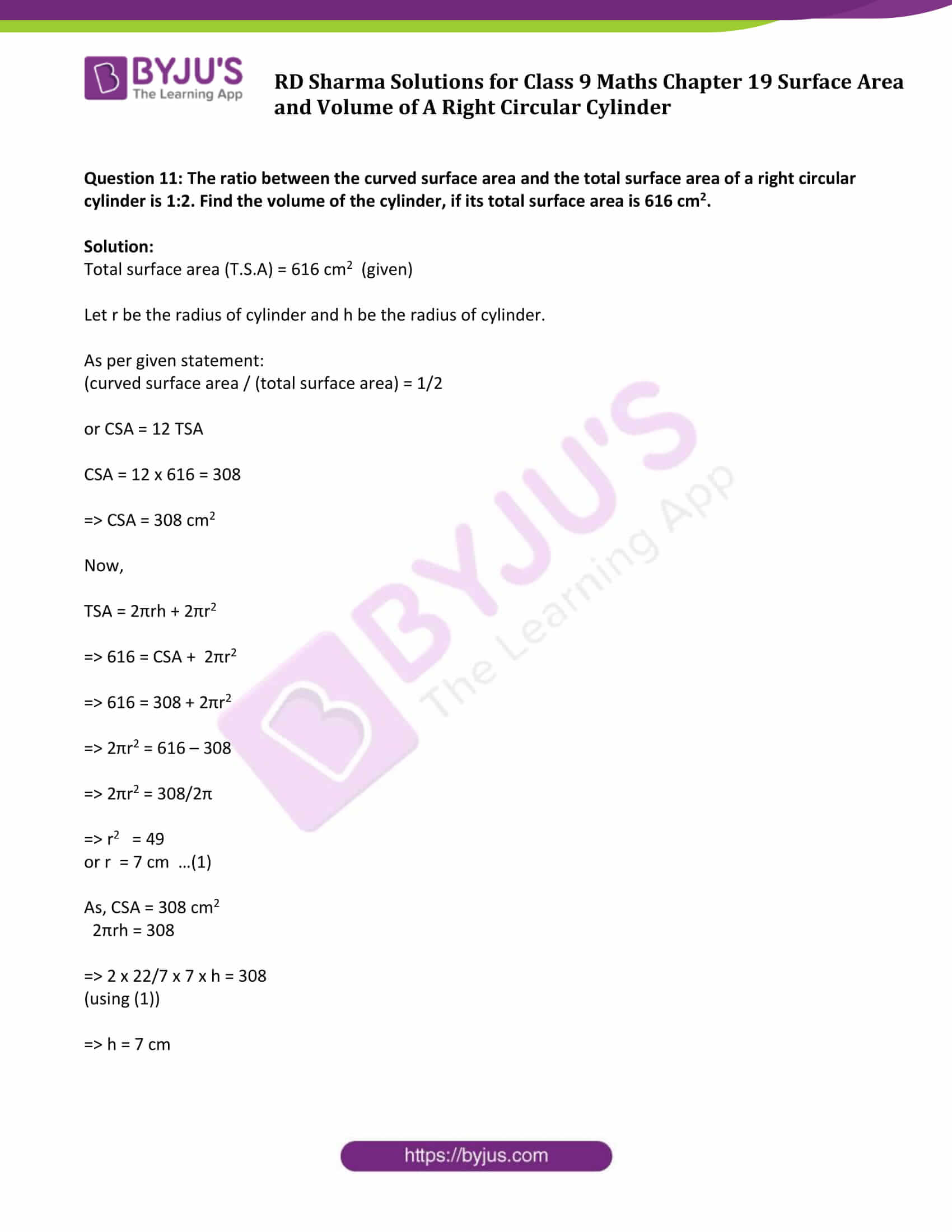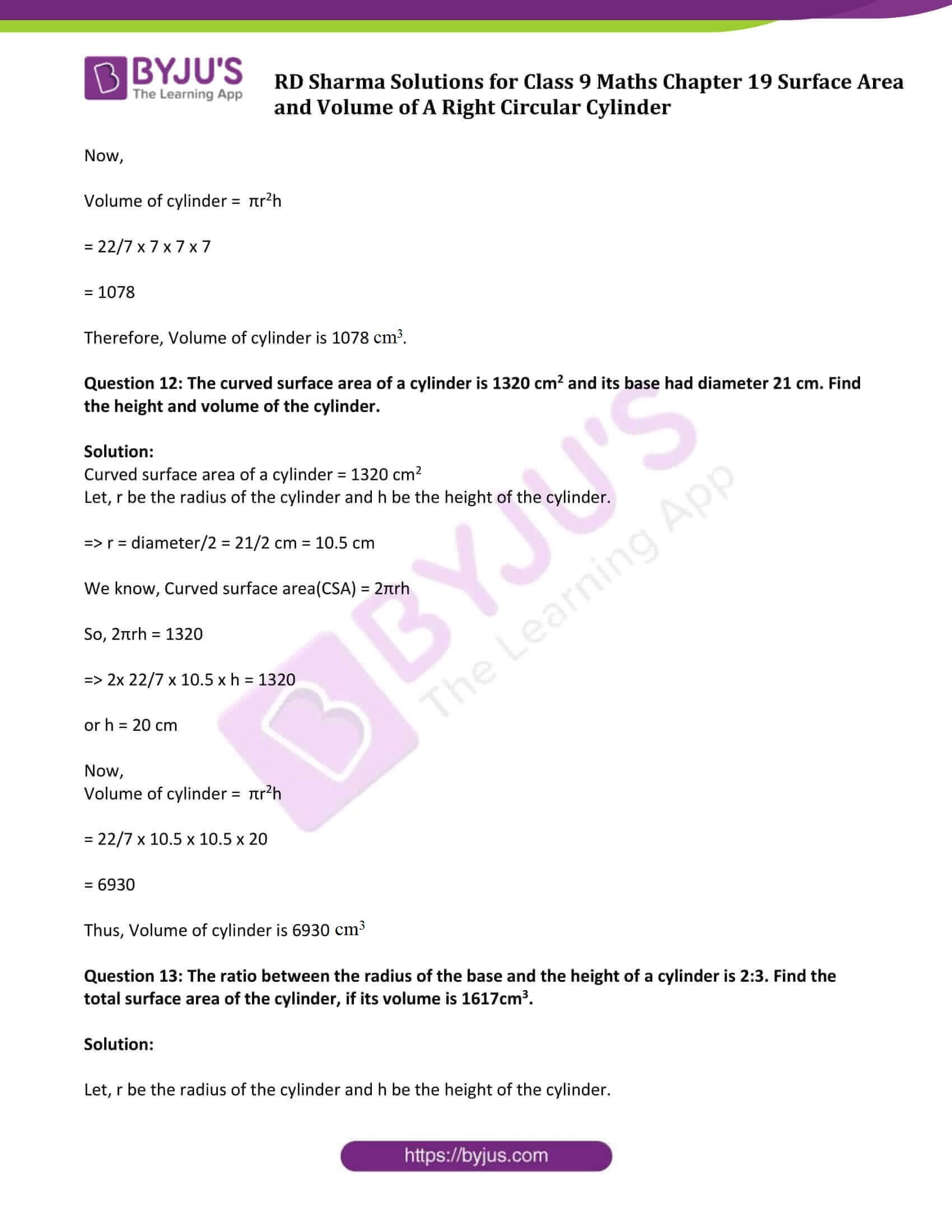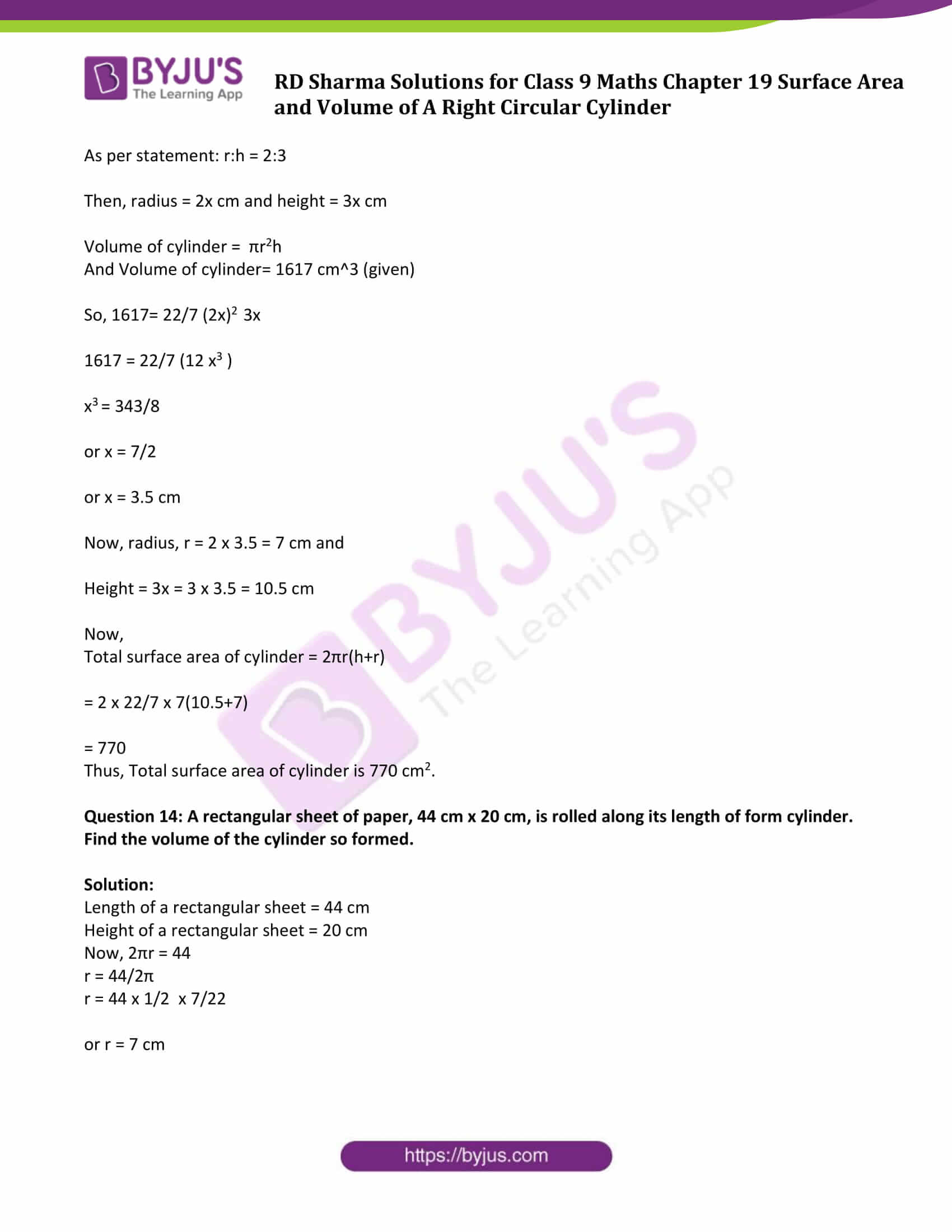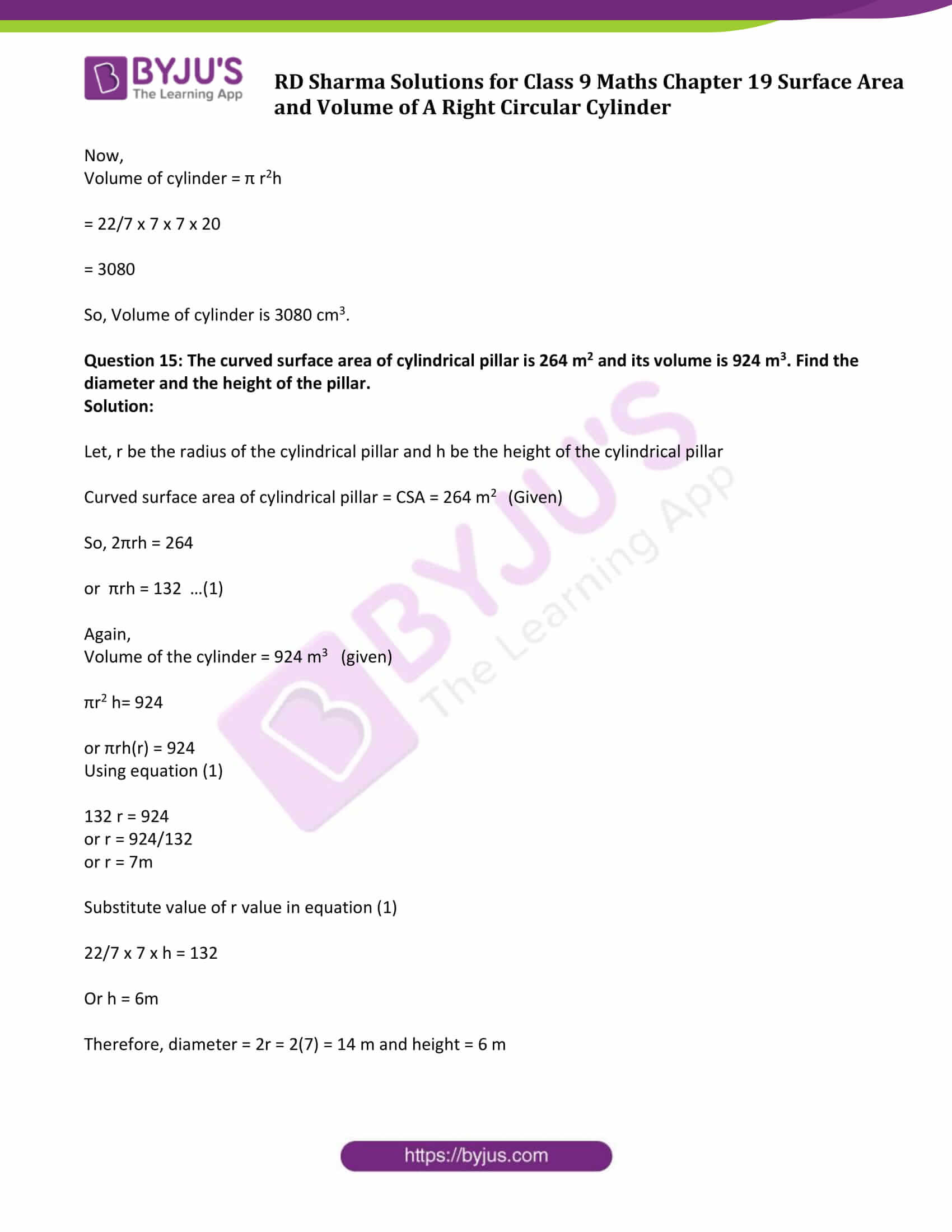### Access Answers to Maths RD Sharma Solutions for Class 9 Chapter 19 Surface Area and Volume of A Right Circular Cylinder Exercise 19.2 Page number 19.20

Question 1: A soft drink is available in two packs- (i) a tin can with a rectangular base of length 5 cm and width 4 cm, having a height of 15 cm and (ii) a plastic cylinder with circular base of diameter 7 cm and height 10 cm, Which container has greater capacity and by how much?

Solution:

(i) Dimensions of a cubical tin can:

Length (L) = 5 cm

Height (H) = 15 cm

Capacity of the tin can = Volume of Tin Can = l x b x h cubic units = (5 x 4 x 15) cm3 = 300 cm3

(ii) Radius of the circular end of the plastic cylinder (R) = diametr/2 = 7/2 cm = 3.5 cm

Height of plastic cylinder (H) = 10 cm

Capacity of plastic cylinder = Volume of cylindrical container = πR2H = 22/7 × (3.5)2 × 10 cm3 = 385 cm3

From (i) and (ii) results, the plastic cylinder has greater capacity.

Difference in capacity = (385 – 300) cm3 = 85 cm3

Question 2: The pillars of a temple are cylindrically shaped. If each pillar has a circular base of radius 20 cm and height 10 m. How much concrete mixture would be required to build 14 such pillars?

Solution:

In this case, we have to find the volume of the cylinders.

Given:

Radius of the base of a cylinder = 20 cm

Height of cylinder = 10 m = 1000 cm

[1m = 100 cm]

Volume of the cylindrical pillar = πR2H

= (22/7×202×1000) cm3

= 8800000/7 cm3 or 8.87 m3

Therefore, volume of 14 pillars = 14 x 8.87 m3 = 17.6 m3

Question 3: The inner diameter of a cylindrical wooden pipe is 24 cm and its outer diameter is 28 cm. The length of the pipe is 35 cm. Find the mass of the pipe, if 1 cm3 of wood has a mass of 0.6 gm.

Solution:

Let r and R be the inner and outer radii of cylindrical pipe.

Inner radius of a cylindrical pipe (r) = 24/2 = 12 cm

Outer radius of a cylindrical pipe (R) = 24/2 = 14 cm

Height of pipe (h) = length of pipe = 35 cm

Mass of pipe = volume x density = π(R2 – r2)h

= 22/7(142 – 122)35

= 5720

Mass of pipe is 5720 cm3

Mass of 1 cm3 wood = 0.6 gm (Given)

Therefore, mass of 5720 cm3 wood = 5720 x 0.6 = 3432 gm = 3.432 kg

Question 4: If the lateral surface of a cylinder is 94.2 cm2 and its height is 5 cm, find:

i) radius of its base (ii) volume of the cylinder

[Use π = 3.141]

Solution:

Lateral surface of the cylinder = 94.2 cm2

Height of the cylinder = 5 cm

(i) Lateral surface of the cylinder = 94.2 cm2

2 πrh = 94.2

or 2 x 3.14 x r x 5 = 94.2

or r = 3 cm

(ii) Volume of the cylinder = πr2h

= (3.14 x 32 x 5) cm3

= 141.3 cm3

Question 5: The capacity of a closed cylindrical vessel of height 1 m is 15.4 liters. How many square meters of the metal sheet would be needed to make it?

Solution:

Given, The capacity of a closed cylindrical vessel of height 1 m is 15.4 liters.

Height of the cylindrical vessel = 15.4 litres = 0.0154 m3

[1m3 = 1000 litres]

Let ‘r’ be the radius of the circular ends of the cylinders, then

πr2h = 0.0154 m3

3.14 x r2 x 1 = 0.0154 m3

or r = 0.07 m

Again,

Total surface area of a vessel = 2πr(r+h)

= 2(3.14(0.07)(0.07+1)) m2

= 0.470 m2

Question 6: A patient in a hospital is given soup daily in a cylindrical bowl of diameter 7 cm. If the bowl is filled with soup to a height of 4 cm, how much soup the hospital has to prepare daily to serve 250 patients?

Solution:

Radius of cylindrical bowl (R) = diameter/2 = 7/2 cm = 3.5 cm

Height = 4 cm

Now,

Volume of soup in 1 bowl = πr2h

= 22/7×3.52×4 cm3

= 154 cm3

Volume of soup in 250 bowls = (250 x 154) cm3

= 38500 cm3

= 38.5 liters

Thus, hospital has to prepare 38.5 liters of soup daily in order to serve 250 patients.

Question 7: A hollow garden roller, 63 cm wide with a girth of 440 cm, is made of 4 cm thick iron. Find the volume of the iron.

Solution:

The outer circumference of the roller = 440 cm

Thickness of the roller = 4 cm and

Its height (h) = 63 cm

Let ‘R’ be the external radius and ‘r’ be the inner radius of the roller.

Circumference of roller = 2πR = 440

Or 2πR = 440

2×22/7 x R = 440

or R = 70

And, inner radius ‘r’ is given as

⇒ r = R – 4

⇒ r = 70 – 4

⇒ r = 66

Now, volume of the iron is given as

V = π(R2−r2)h

V = 22/7 (702−662)63

V = 107712

Therefore, required volume is 107712 cm3.

Question 8: A solid cylinder has a total surface area of 231 cm2. Its curved surface area is 2/3 of the total surface area. Find the volume of the cylinder.

Solution:

Total surface area = 231 cm2

As per given statement: Curved surface area = 2/3(Total surface area)

Curved surface area = 2/3 x 231 = 154

So, Curved surface area = 154 cm2 …(1)

We know, Curved surface area of cylinder = 2πrh + 2πr2

Or 2πrh + 2πr2 = 231 …..(2)

Here 2πrh is the curved surface area, so using (1), we have

⇒ 154 + 2πr2 = 231

⇒ 2πr2 = 231- 154

⇒ 2 x 22/7 x r2 = 77

⇒ r2 = 49/4

or r = 7/2

Find the value of h:

CSA = 154 cm2

⇒ 2πrh = 154

⇒ 2 x 22/7 x 7/2 x h = 154

⇒ h = 154/22

⇒ h = 7

Now,

Find Volume of the cylinder:

V = πr2h

= 22/7 x 7/2 x 7/2 x 7

= 269.5

The volume of the cylinder is 269.5 cm3

Question 9: The cost of painting the total outside surface of a closed cylindrical oil tank at 50 paise per square decimetre is Rs 198. The height of the tank is 6 times the radius of the base of the tank. Find the volume corrected to 2 decimal places.

Solution:

Let ‘r’ be the radius of the tank.

As per given statement: Height (h) = 6(Radius) = 6r dm

Cost of painting for 50 paisa or Rs 1/2 per dm2 = Rs 198 (Given)

⇒ 2πr(r+h) × 1/2 = 198

⇒ 2×22/7×r(r+6r) × 1/2 = 198

⇒ r = 3 dm

And, h = (6 x 3) dm = 18 dm

Now,

Volume of the tank = πr2h = 22/7×9×18 = 509.14 dm3

Question 10: The radii of two cylinders are in the ratio 2 : 3 and their heights are in the ratio 5 : 3. Calculate the ratio of their volumes and the ratio of their curved surfaces.

Solution:

Let the radius of the cylinders be 2x and 3x and the height of the cylinders be 5y and 3y.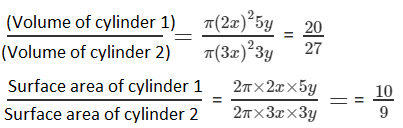Question 11: The ratio between the curved surface area and the total surface area of a right circular cylinder is 1:2. Find the volume of the cylinder, if its total surface area is 616 cm2.

Solution:

Total surface area (T.S.A) = 616 cm2 (given)

Let r be the radius of cylinder and h be the radius of cylinder.

As per given statement:

(curved surface area / (total surface area) = 1/2

or CSA = 12 TSA

CSA = 12 x 616 = 308

⇒ CSA = 308 cm2

Now,

TSA = 2πrh + 2πr2

⇒ 616 = CSA + 2πr2

⇒ 616 = 308 + 2πr2

⇒ 2πr2 = 616 – 308

⇒ 2πr2 = 308/2π

⇒ r2 = 49

or r = 7 cm …(1)

As, CSA = 308 cm2

2πrh = 308

⇒ 2 x 22/7 x 7 x h = 308

(using (1))

⇒ h = 7 cm

Now,

Volume of cylinder = πr2h

= 22/7 x 7 x 7 x 7

= 1078

Therefore, Volume of cylinder is 1078 cm3.

Question 12: The curved surface area of a cylinder is 1320 cm2 and its base had diameter 21 cm. Find the height and volume of the cylinder.

Solution:

Curved surface area of a cylinder = 1320 cm2

Let, r be the radius of the cylinder and h be the height of the cylinder.

⇒ r = diameter/2 = 21/2 cm = 10.5 cm

We know, Curved surface area(CSA) = 2πrh

So, 2πrh = 1320

⇒ 2x 22/7 x 10.5 x h = 1320

or h = 20 cm

Now,

Volume of cylinder = πr2h

= 22/7 x 10.5 x 10.5 x 20

= 6930

Thus, Volume of cylinder is 6930 cm3.

Question 13: The ratio between the radius of the base and the height of a cylinder is 2:3. Find the total surface area of the cylinder, if its volume is 1617cm3.

Solution:

Let, r be the radius of the cylinder and h be the height of the cylinder.

As per statement: r:h = 2:3

Then, radius = 2x cm and height = 3x cm

Volume of cylinder = πr2h

And Volume of cylinder= 1617 cm^3 (given)

So, 1617= 22/7 (2x)2 3x

1617 = 22/7 (12 x3 )

x3 = 343/8

or x = 7/2

or x = 3.5 cm

Now, radius, r = 2 x 3.5 = 7 cm and

Height = 3x = 3 x 3.5 = 10.5 cm

Now,

Total surface area of cylinder = 2πr(h+r)

= 2 x 22/7 x 7(10.5+7)

= 770

Thus, Total surface area of cylinder is 770 cm2.

Question 14: A rectangular sheet of paper, 44 cm x 20 cm, is rolled along its length of form cylinder. Find the volume of the cylinder so formed.

Solution:

Length of a rectangular sheet = 44 cm

Height of a rectangular sheet = 20 cm

Now, 2πr = 44

r = 44/2π

r = 44 x 1/2 x 7/22

or r = 7 cm

Now,

Volume of cylinder = π r2h

= 22/7 x 7 x 7 x 20

= 3080

So, Volume of cylinder is 3080 cm3.

Question 15: The curved surface area of cylindrical pillar is 264 m2 and its volume is 924 m3. Find the diameter and the height of the pillar.

Solution:

Let, r be the radius of the cylindrical pillar and h be the height of the cylindrical pillar

Curved surface area of cylindrical pillar = CSA = 264 m2 (Given)

So, 2πrh = 264

or πrh = 132 …(1)

Again,

Volume of the cylinder = 924 m3 (given)

πr2 h= 924

or πrh(r) = 924

Using equation (1)

132 r = 924

or r = 924/132

or r = 7m

Substitute value of r value in equation (1)

22/7 x 7 x h = 132

Or h = 6m

Therefore, diameter = 2r = 2(7) = 14 m and height = 6 m

## RD Sharma Solutions for Class 9 Maths Chapter 19 Surface Area and Volume of A Right Circular Cylinder Exercise 19.2

RD Sharma Solutions Class 9 Maths Chapter 19 Surface Area and Volume of A Right Circular Cylinder Exercise 19.2 is based on following topics:

• Volume of a Cylinder
• Volume of a Hollow Cylinder